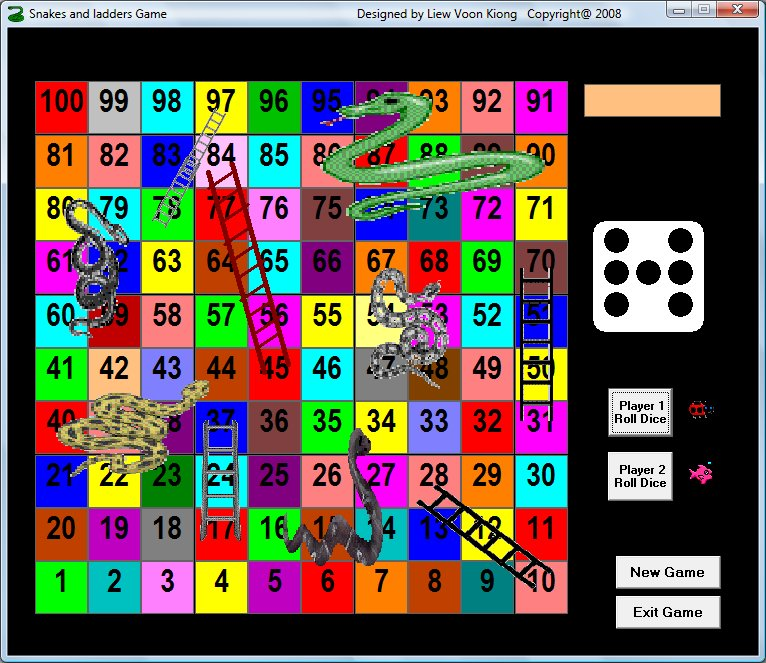Snakes and Ladders game is a popular board game for young children. This game usually involves two or more players and they take turns to move by rolling a dice. On the way to the finishing point, the players will meet with some hurdles in the form of snakes and some opportunities in the form of ladders. Whenever the player encounters a snake (or more accurately, the snake's head), he or she will be thrown back to an earlier position (which is at the snake's tail). On the other hand, whenever the player encounters a ladder, he or she can climb up the ladder to a higher position. The player who reaches the finishing point first wins the game

To design the program, we use mathematical logics. Since the board comprises 10 rows and 10 columns, each box thus represents a cell with the coordinate  (column, row). Therefore, we need to define the coordinate of every cell by declaring two arrays row(10) and col(10) at the beginning of the procedure. The initial position for our design is c(1)=600 and r(1)=8200, yours may be different. To assign the column and row coordinates to all the boxes, we use the following For...Next loop:

For i = 1 To 9
c(i + 1) = c(i) + 800
Next

To move the player's piece, we employed the method object.move  col(i), row(j), where we initiate the values of col(i) and row(j) in a For....Next loop. As the motion is in a zigzag manner, we need to control the motion using reverse order and by imposing some conditions. Here we list some parts the code:

'To declare the variables

Option Base 1

Dim c(10) As Variant
Dim r(10) As Variant
Dim x As Integer
Dim m As Integer
Dim n As Integer
Dim num As Integer
Dim totalnum As Single
Dim totalnum1 As Single
Dim player As Integer
Dim t As Integer

'To assign the column and row coordinates to all the boxes

c(1) = 600
r(1) = 8200
For i = 1 To 9
c(i + 1) = c(i) + 800
Next
For j = 1 To 9
r(j + 1) = r(j) - 800
Next
End Sub

To move a player's piece to the original position

Private Sub Command2_Click()
'
Image1(0).Move 10200, 5520
Image1(1).Move 10200, 6480
totalnum = 0
totalnum1 = 0

End Sub

#### The Interface#### The Video Demo

View Complete Code.